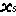# Components of an equation

Expressions can consist of one or more of these components: numerical constants, symbolic names, mathematical operators, functions, and conditional expressions.

## Numerical constants

Numerical constants are any numeric values, consisting of the digits 0-9, with an optional decimal point and a leading minus sign (for negative values). A leading 0 is optional for numbers less than 1.0. Standard scientific notation is provided (e.g. 0.12345E3 for 123.45), or you can enter very small or very large numbers by multiplying a value by 10 raised to a power, e.g. 0.12345*10^11 or 0.12345*10^-11. See also the section on Arrays and lists for details of how to enter arrays of numerical constants.

## Symbolic names

A symbolic name represents the value of one of the variables, compartments or flows that influences the element in question. Each time the value of the expression is calculated, the current value of the influencing element is used.

The only symbolic names for model quantities that you can enter into an expression are those that influence the element in question. These are listed by clicking on thepull-down menu button on the equation bar, and in the "Parameter" column of the main tab of the equation dialogue box. These must be entered exactly as shown, using the same punctuation (note especially the _ underscore symbols), and using the same case. The easiest way to do this is to select them from the pull-down menu, or to double-click on the name in the "Parameter" column.

Note that the symbolic name entered into the expression is the local name. This can be different from the name displayed on the model diagram, either to make the expression easier to read, or to avoid using certain illegal characters. Local names will also be modified when values come from a multi-instance submodel.

## Mathematical operators

You can use the following mathematical operators:

 + e.g. 15+7 (evaluates to 22) - e.g. 15-7 (evaluates to 8) * e.g. 15*7 (evaluates to 105) / e.g. 15/7 (evaluates to 2.1428…) // integer division e.g. 15//7 (evaluates to 2) % integer modulo e.g. 94%22 (evaluates to 6) ^ raise to a power e.g. 15^2 (evaluates to 225)

## Functions

Simile provides a large number of functions that you can use in expressions already built-in, and the facility to extend this list.

## Conditional expressions

Equations can include conditional expressions. These enable a complex expression to be constructed from a number of sub-expressions, with the conditional elements being used to select between one expression or another. Standard Boolean operators are available for constructing conditional expressions.

## Intermediate variables

The equation dialogue box can be used to enter one or more assignments, before the expression that returns the element's value. These intermediate variables can be used to simplify complex expressions.